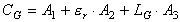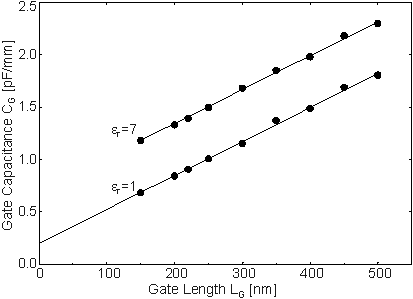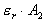Next: 6.2.3.3 Dependence of CG on Passivation Thickness Up: 6.2.3 RF Characteristics Previous: 6.2.3.1 Reduction of the Gate Length

6.2.3.2 Contributions to the Gate Capacitance

In order to identify the different contributions to CG, we use the procedure described in Section 6.1.2. The capacitive coupling of the gate metal to the ohmic contacts and to the semiconductor material is minimized by setting er1 = er2 = er = 1 (no passivation at all). The opposite extreme is represented by the case er = 7.  Figure 6.32 shows the simulated CG as a function of LG for both cases. Again a linear fit can be found for CG versus LG and also on er which was demonstrated in Section 6.1.2:. (62)Figure 6.32 Simulated gate capacitance CG vs. gate length LG for two different er at VDS = 2.0 V, ID = 160 mA/mm.

By extrapolation of the straight line for er = 1 to LG = 0, the fringe capacitance CF = A1 + A2 is obtained. In Figure 6.32, CF  200 fF/mm. From the separation of the two straight lines A2 can be deduced. In this case, A2 » 85 fF/mm. Finally, A3 = 3.2 nF/mm2 is given by the slope of the two lines. (for a gate with LG = 100 nm, this is equivalent to 320 fF/mm).

A1 and A3 depend on the epitaxial layers, whereasis given by the geometry of the contacts and the permittivity of the dielectric. In the following the influence ofon the thickness of the passivation and the shape of the T­gate will be investigated.Next: 6.2.3.3 Dependence of CG on Passivation Thickness Up: 6.2.3 RF Characteristics Previous: 6.2.3.1 Reduction of the Gate Length

Helmut Brech
1998-03-11